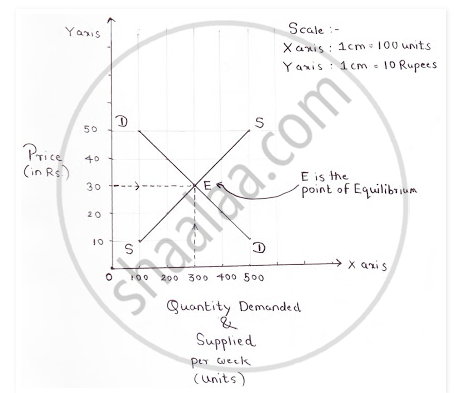# Determination of Equilibrium Price Under Perfect Competition. - Economics

Determination of equilibrium price under perfect competition.

#### Solution

Equilibrium price is the price at which quantity demanded is equal to quantity supplied. The price of the product under perfect competition, is influenced by both buyers and sellers and equilibrium price is determined by the interaction of demand and supply forces. Under Perfect competition an equilibrium price is determined by the intersection of market demand and market supply as shown in the following diagram.Concept: Equilibrium Price
Is there an error in this question or solution?
2014-2015 (March)

Share